Next: Repetition of Information Up: Desirable Properties of Decomposition Previous: Lossless-Join Decomposition

### Dependency Preservation

1. Another desirable property in database design is dependency preservation.
• We would like to check easily that updates to the database do not result in illegal relations being created.
• It would be nice if our design allowed us to check updates without having to compute natural joins.
• To know whether joins must be computed, we need to determine what functional dependencies may be tested by checking each relation individually.
• Let F be a set of functional dependencies on schema R.
• Let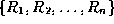be a decomposition of R.
• The restriction of F to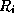is the set of all functional dependencies in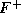that include only attributes of.
• Functional dependencies in a restriction can be tested in one relation, as they involve attributes in one relation schema.
• The set of restrictions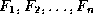is the set of dependencies that can be checked efficiently.
• We need to know whether testing only the restrictions is sufficient.
• Let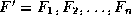.
• F' is a set of functional dependencies on schema R, but in general,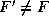.
• However, it may be that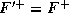.
• If this is so, then every functional dependency in F is implied by F', and if F' is satisfied, then F must also be satisfied.
• A decomposition having the property thatis a dependency-preserving decomposition.
2. The algorithm for testing dependency preservation follows this method:

``` computefor each schemain D do

begin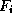:= the restriction ofto;

end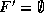for each restrictiondo

begin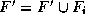end

compute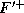;

if () then return (true)

else return (false);

```

3. We can now show that our decomposition of Lending-schema is dependency preserving.
• The functional dependency

``` bnameassets bcity

```

can be tested in one relation on Branch-schema.

• The functional dependency

``` loan#amount bname

```

can be tested in Loan-schema.

4. As the above example shows, it is often easier not to apply the algorithm shown to test dependency preservation, as computingtakes exponential time.
5. An Easier Way To Test For Dependency Preservation

Really we only need to know whether the functional dependencies in F and not in F' are implied by those in F'.

In other words, are the functional dependencies not easily checkable logically implied by those that are?

Rather than computeand, and see whether they are equal, we can do this:

• Find F - F', the functional dependencies not checkable in one relation.
• See whether this set is obtainable from F' by using Armstrong's Axioms.
• This should take a great deal less work, as we have (usually) just a few functional dependencies to work on.
Use this simpler method on exams and assignments (unless you have exponential time available to you).Next: Repetition of Information Up: Desirable Properties of Decomposition Previous: Lossless-Join Decomposition

Osmar Zaiane
Thu Jun 18 12:56:34 PDT 1998